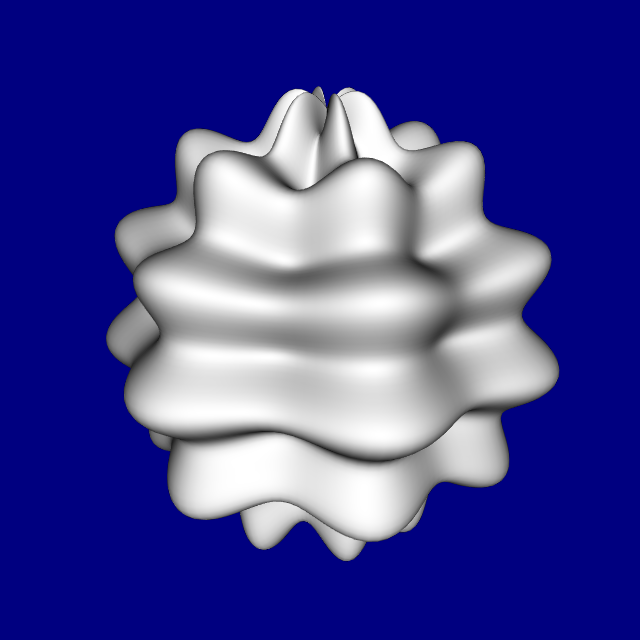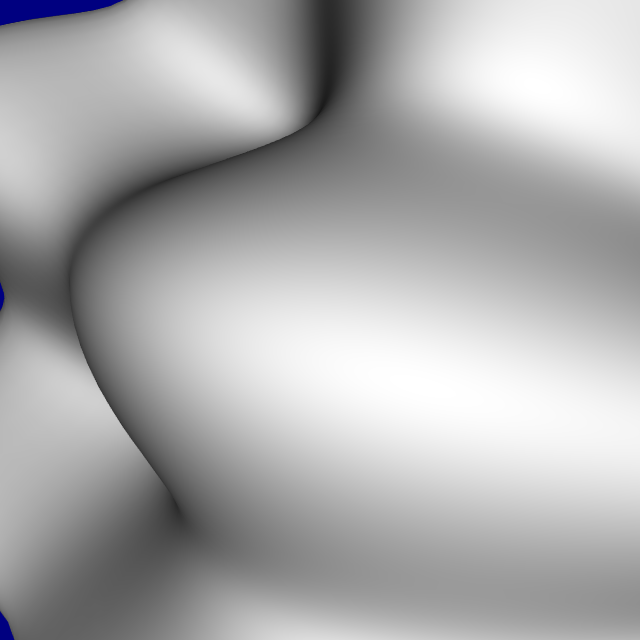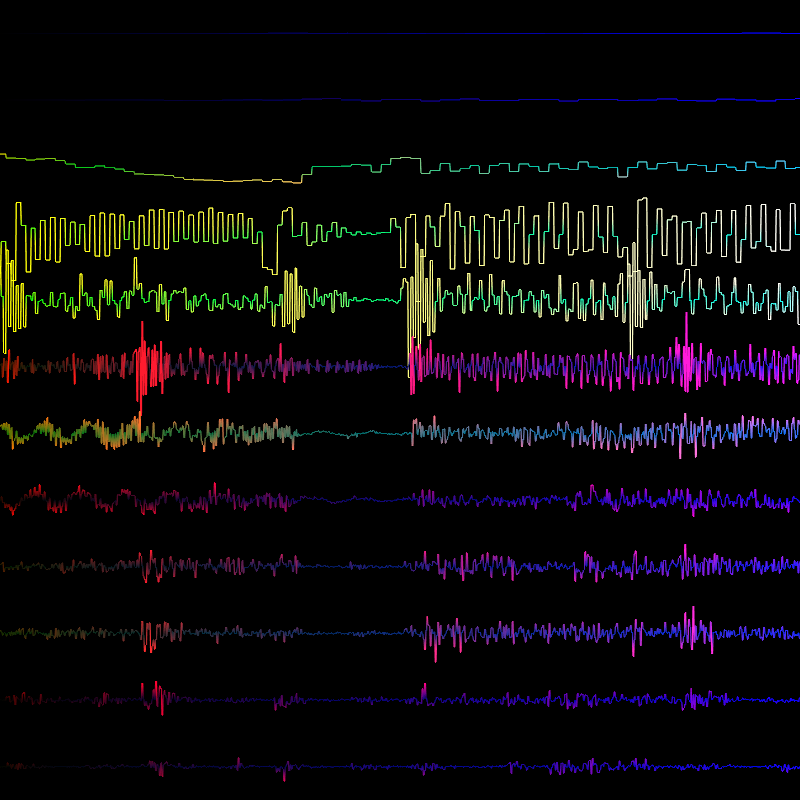# GPU Experiments

A blog which discusses various GPU applications including visualization, GPGPU and games.

## Sunday, January 10, 2010

### More tessellator fun

This is just a quick update post on projects I am currently working on.

1. Adding support for the tessellator into my realtime parametric surface plotting program. The original version of this program simply rendered an equally distributed mesh and offset the vertices in the vertex shader. Now I am tessellating patches in realtime to determine how many triangles should be output.

The initial results are promising. Shown here are three images, each of the same plot but with a differing camera position.2. Music visualization program using wavelets. I plan on bringing the tessellator into this application once I go 3D.## Sunday, January 3, 2010

### Integer division

Beware integer division on the GPU.

In a certain compute shader I am working on, this code
``if (tcoord.x < 0) tcoord.x += gridsize.x;if (tcoord.y < 0) tcoord.y += gridsize.y;if (tcoord.z < 0) tcoord.z += gridsize.z;if (tcoord.x >= gridsize.x) tcoord.x -= gridsize.x;if (tcoord.y >= gridsize.y) tcoord.y -= gridsize.y;if (tcoord.z >= gridsize.z) tcoord.z -= gridsize.z;``

runs much faster than using
``tcoord = (tcoord + gridsize) % gridsize;``